Latest Banking jobs   »   Quantitative Aptitude Quiz For ESIC- UDC,...

# Quantitative Aptitude Quiz For ESIC- UDC, Steno, MTS Prelims 2022-5th January

Directions (1-5): In the following questions two quantities are given for each question. Compare the numeric value of both the quantities and answers accordingly.

Q1. A certain sum of money amounts to Rs. 2613 in 6 years at 5% per annum.
Quantity I: No. of years in which the same amount becomes Rs. 3015 at same rate.
Quantity II: 11 years.
(a) Quantity I > Quantity II
(b) Quantity I < Quantity II
(c) Quantity I ≥ Quantity II
(d) Quantity I ≤ Quantity II
(e) Quantity I = Quantity II or No relation

Q2. Quantity I : A sphere of diameter 13.4 cm is melted and cast into a right circular cone of height 26.8 cm. The radius of the base of the cone is ?
Quantity II: 5.95 cm.
(a) Quantity I > Quantity II
(b) Quantity II > Quantity I
(c) Quantity I ≥ Quantity II
(d) Quantity II ≥ Quantity I
(e) Quantity I = Quantity II or relation can’t be established.

Q3. A, B and C complete a task and receive Rs. 8880. They work for equal number of days. Five times of A’s efficiency is equal to four times of B’s efficiency which is equal to six times of C’s efficiency.
Quantity I: 2400
Quantity II: Amount received by C.
(a) Quantity I > Quantity II
(b) Quantity I < Quantity II
(c) Quantity I ≥ Quantity II
(d) Quantity I ≤ Quantity II
(e) Quantity I = Quantity II or No relation

Q4. A solution of acid and water contains 20% acid in it.
Quantity I: Percentage of water that must be evaporated from solution to get a 50% acid solution.
Quantity II: 80%
(a) Quantity I > Quantity II
(b) Quantity I < Quantity II
(c) Quantity I ≥ Quantity II
(d) Quantity I ≤ Quantity II
(e) Quantity I = Quantity II or No relation

Q5. A person lends ⅓rd of his money at 15% while the rest at 18% per annum rate at simple interest.
Quantity I: His annual rate of interest on the whole sum.
Quantity II: 16%.
(a) Quantity I > Quantity II
(b) Quantity I < Quantity II
(c) Quantity I ≥ Quantity II
(d) Quantity I ≤ Quantity II
(e) Quantity I = Quantity II or No relation

Q6. Ravi sent ‘X’ messages in a day, 20% in which sent to Ram. Out of remaining 25% sent to Shyam. Remaining messages sent to Rahul and Gopal is in the ratio 4 : 5. Rahul got 24 message.
Quantity I: Value of ‘1.5X’
Quantity II: ‘X + 35’
(a) Quantity I > Quantity II
(b) Quantity I < Quantity II
(c) Quantity I ≥ Quantity II
(d) Quantity I ≤ Quantity II
(e) Quantity I = Quantity II or No relation

Q7. Area of a square of side 16 cm is same as area of a rectangle whose breadth is 8 cm.
Quantity I: Perimeter of square.
Quantity II: Perimeter of rectangle.
(a) Quantity I > Quantity II
(b) Quantity I < Quantity II
(c) Quantity I ≥ Quantity II
(d) Quantity I ≤ Quantity II
(e) Quantity I = Quantity II or No relation

Q8. Train ‘A’ can cross a pole in 20 seconds and a platform which is 60 m long in 25 seconds. Train ‘A’ can cross train ‘B’ coming from opposite direction in 15 seconds. Ratio between speed of train ‘A’ to train ‘B’ is 2 : 3.
Quantity I: Length of train ‘B’
Quantity II: Length of train ‘A’
(a) Quantity I > Quantity II
(b) Quantity I < Quantity II
(c) Quantity I ≥ Quantity II
(d) Quantity I ≤ Quantity II
(e) Quantity I = Quantity II or No relation

Q9. Probability of selecting one yellow ball out of a box consisting some yellow balls and some green balls is 4/7.
Quantity I: No. of yellow balls in box.
Quantity II: No. of green balls in box.
(a) Quantity I > Quantity II
(b) Quantity I < Quantity II
(c) Quantity I ≥ Quantity II
(d) Quantity I ≤ Quantity II
(e) Quantity I = Quantity II or No relation

Q10. Quantity I : Distance covered in upstream in 10.8 hours
Ratio of upstream speed to downstream speed is 1 : 11. If speed of boat in still water is 30 km/hr.
Quantity II : Find x.
The time taken by a boat for covering ‘x – 18’ km upstream is equal to time taken by it for covering ‘x’ km downstream. Upstream speed is 6 km/hr less than downstream speed and speed of boat in still water in 15 kmph.
(a) Quantity I > Quantity II
(b) Quantity II > Quantity I
(c) Quantity I ≥ Quantity II
(d) Quantity II ≥ Quantity I
(e) Quantity I = Quantity II or relation can’t be established

Directions (11-15): In the following questions two quantities are given for each question. Compare the numeric value of both the quantities and answers accordingly

Q11. Ram invested Rs80000 for a year and Shyam invested Rs72000 For 8 months.
Quantity I : If total profit at the end of year is Rs11400 then the share of Ram.
Quantity II : Rs. 1420
(a) Quantity I > Quantity II
(b) Quantity II > Quantity I
(c) Quantity I ≥ Quantity II
(d) Quantity II ≥ Quantity I
(e) Quantity I = Quantity II or relation can’t be established

Q12. 24 men can do a work in 20 days and 36 women can do the same work in 24 days
Quantity I : Time taken by 12 man to complete whole work
Quantity II : Time taken by 16 women to complete whole work.
(a) Quantity I > Quantity II
(b) Quantity II > Quantity I
(c) Quantity I ≥ Quantity II
(d) Quantity II ≥ Quantity I
(e) Quantity I = Quantity II or relation can’t be established

Q13. Average age of A, B and C is 37 years and average age of A and B is 23 years
Quantity I : What is the age of C
Quantity II : 65 years
(a) Quantity I > Quantity II
(b) Quantity II > Quantity I
(c) Quantity I ≥ Quantity II
(d) Quantity II ≥ Quantity I
(e) Quantity I = Quantity II or relation can’t be established

Q14. A train of length 360 m is travelling at a speed of 54 km/hr
Quantity I : Find the time take by train to cross a pole
Quantity II : If its speed is increased by 16⅔% then find the time in which it can cross a platform of length 130 m.
(a) Quantity I > Quantity II
(b) Quantity II > Quantity I
(c) Quantity I ≥ Quantity II
(d) Quantity II ≥ Quantity I
(e) Quantity I = Quantity II or relation can’t be established

Q15. Quantity I : A sphere of diameter 13.4 cm is melted and cast into a right circular cone of height 26.8 cm. The radius of the base of the cone is ?
Quantity II: 5.95 cm.
(a) Quantity I > Quantity II
(b) Quantity II > Quantity I
(c) Quantity I ≥ Quantity II
(d) Quantity II ≥ Quantity I
(e) Quantity I = Quantity II or relation can’t be established

Solutions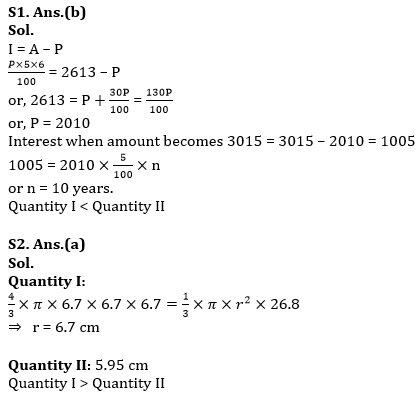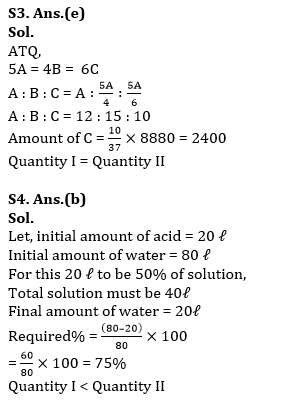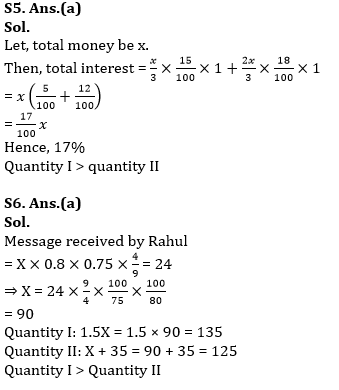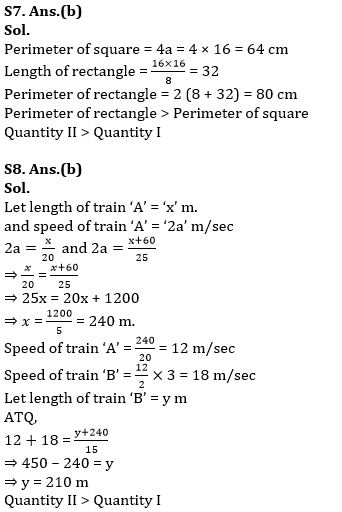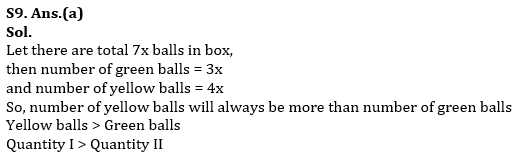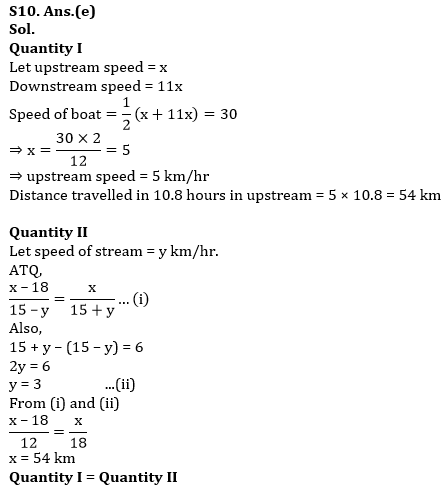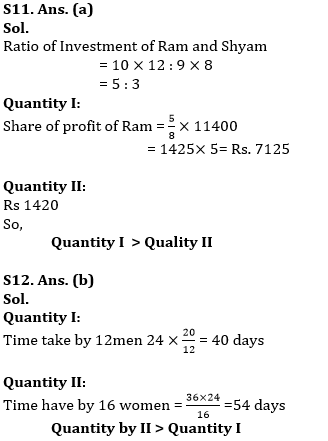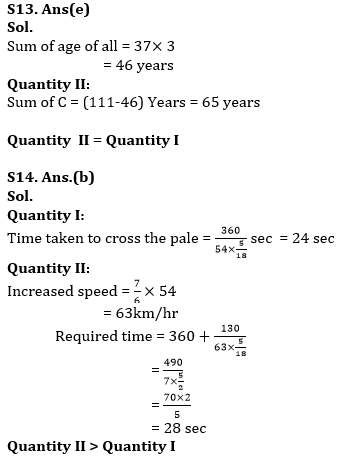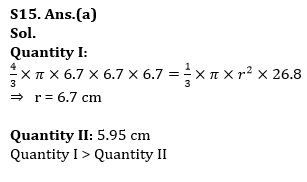#### Congratulations!Union Budget 2023-24: Free PDF